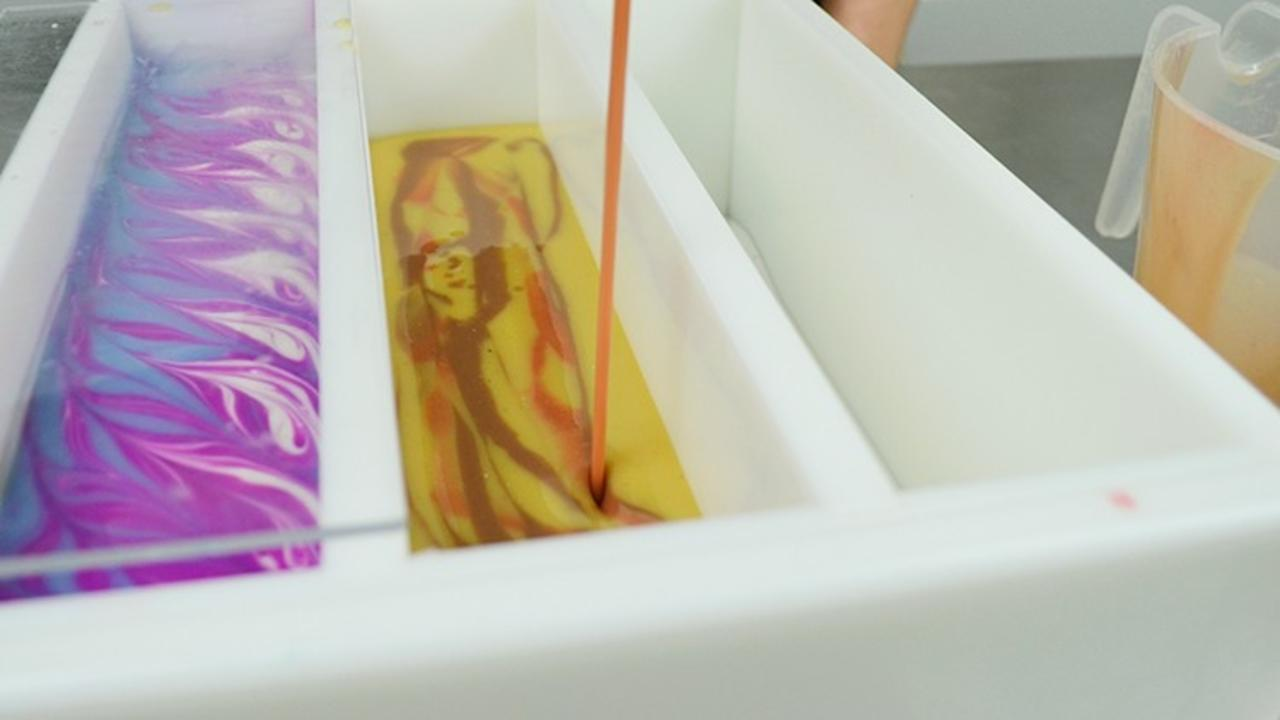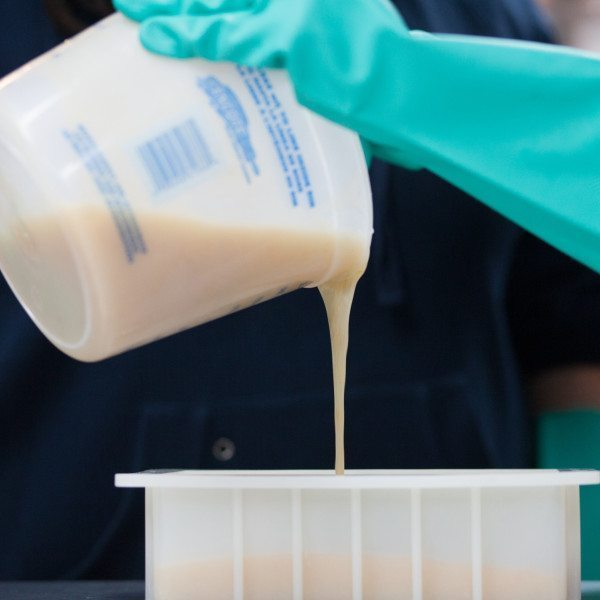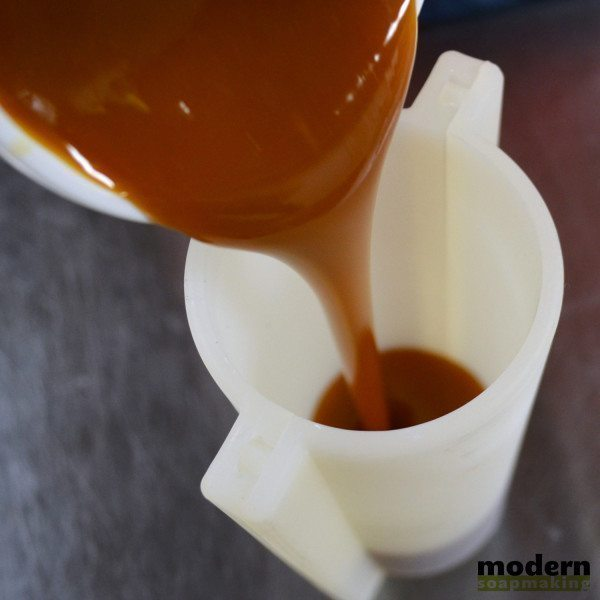# How to Resize a Soap Recipe for Your Mold (U.S. Standard and Metric!)

One of the most common questions I receive is how to resize a soap recipe here on Modern Soapmaking to fit a different mold than what is used in a tutorial. To resize a soap recipe, we first need to know how much soap or oil your mold will hold by calculating your mold capacity.

There are two calculations that are widely accepted by soapmakers to determine the amount of oils needed for a recipe to fit a mold. These calculations are based on determining the volume of a soap mold and then finding the amount of the volume that belongs to the oils themselves.

The U.S. Standard (United States Soapmakers) Calculation:

Length of Soap Mold in Inches x Width of Soap Mold in Inches x Depth of Soap Pour in Inches x .40 or .38 = Amount of Oils Needed in Ounces

The Metric (International Soapmakers) Calculation:

Length of Soap Mold in Centimeters x Width of Soap Mold in Centimeters x Depth of Soap Pour in Centimeters x .70 or .65 = Amount of Oils in Grams

Now, these calculations are based on using close to full water (or full water if using .38 or .65 instead) in a soap recipe, so if you use a water discount, the final calculations won't be as accurate for you. You can easily modify these calculations for your water discount by customizing your calculations.Before you pour, know how much soap you really need!

Let's first run through where I think the .40/.38 or .70/.65 comes from:

The .40/.38 or .70/.65 appears to come from calculating the individual volume amounts for each ingredient and determining the amount of the total that belongs to the oils. So, to start, we need to know the density of our oils, the lye, and our water. The density is nearly the same numerically as the specific gravity, which you can usually find on an ingredients MSDS (Material Safety Data Sheet) which your supplier should have on hand and available to you.

The density of most oils is 0.900 to 0.920 grams per cubic centimeter. For instance, a specific variety of olive oil I have on hand is 0.918 grams per cubic centimeter. The density of  lye is 2.13 grams per cubic centimeter, and the density of water is 1 gram per cubic centimeter.

To find out what our multiplier is (the .40 or .70) for our recipe, we need to find the split of volume per ingredient. Let's say that the base formula we always use is 100% olive oil soap, with a 7% superfat, and 40% lye concentration.

You'll need to pick a random oil weight - I'm using 1078 grams (roughly 38 ounces) which is usually around the amount of a Bramble Berry 10" mold. You can use 16 ounces (454 grams) if you'd like - this number doesn't matter, we just need to know what amount of the total volume of a supposed recipe is the oil itself.

If we throw our recipe into Soap Calc, we know that for a 7% superfat and 40% lye solution of 1078 grams of olive oil gives us the following water and lye amounts:

Water = 203.72 grams

Lye = 135.82 grams

So, we have these amounts of each ingredient, which we will divide by the density of the material to find the volume of each:

Olive Oil = 1078 grams / 0.918 grams per cubic centimeter = 1174.29 cubic centimeters

Water = 203.72 grams / 1 grams per cubic centimeter = 203.72 cubic centimeters

Lye = 135.82 grams / 2.13 grams per cubic centimeter = 63.77 cubic centimeters

If we add the individual volume of each ingredient (1174.29 + 203.72 + 63.77), we find that we have a total volume of 1441.78 cubic centimeters.

From here, we can determine the amount of the volume dedicated to the olive oil since we know the total volume of the formula and the amount of the olive oil alone.

1078 grams of Olive Oil / 1441.78 cubic centimeters = .748

So, a soapmaker using this formula and the metric calculation would use .748 instead of .70 to reach a more accurate calculation of the amount of olive oil they would need for a formula.

Need to go U.S. Standard? Follow the metric calculations and convert to U.S. standard:

1078 grams converts to 38.03 ounces, and 1441.78 cubic centimeters converts to 87.98 cubic inches.

38.03 ounces of Olive Oil / 87.98 cubic inches = .432

An U.S. standard measurement using soapmaker (aka a soapmaker in the United States ) would use .432 for their calculation instead of .40.

How accurate is the new calculation?

This is still slightly off since we rounded a lot throughout our math, and also because we do need a waste allowance (the smidgen of soap left in our soap pot after pouring) so I recommend rounding the hundredth place digit up by one. Doing so would give the metric soapmaker a 0.75 and the U.S standard soapmaker a 0.44 in the above calculation and formula.

How do I calculate for my specific formula?

You'll need to know the density of each oil you use, and then replace the olive oil part of the calculation with each oil individually. You can even take this a step further and hand-calculate saponification values and specific volume of each oil during saponification, but I think that's just a bit too much. (I believe this is how the Soapmaker software determines recipe resizing per mold, but I'm not 100% sure.)

For example, I used olive oil once again, but changed the lye solution strength and amount of example olive oil used to 500 grams, and these are the metric & U.S. standard calculation numbers, rounded up:

100% olive oil soap with 25% lye solution, 7% superfat = .66 metric calculation -OR- .38 U.S. standard calculation

100% olive oil soap with 33% lye solution, 7% superfat = .72 metric calculation -OR- .42 U.S. standard calculation

100% olive oil soap with 40% lye solution, 7% superfat = .75 metric calculation -OR- .44 U.S. standard calculation

If you don't want to figure the calculations for your own recipe, using the above modified numbers will get you a bit closer than the default standard of .40 or .70 but it's best to find the full calculation for your oil profile.

A recap on how to use these multipliers as an example:

Let's say you are using a 100% olive oil recipe with a 33% lye solution and 7% superfat. From above, we know that our multiplier is .72 for metric or .42 for U.S. standard.

We measure the interior of our mold (that's where the soap goes!) and find that our measurements are as follows:

Length of mold: 10 inches / 25.4 centimeters

Width of mold: 2.5 inches / 6.35 centimeters

Depth of pour: 3.5 inches / 8.89 centimeters

*A note on depth of pour: most soapmakers do not fill their soap mold to the brim. Your depth of pour is going to be determined by how tall you want the soap to be that fits in the mold. For instance, if a mold is 4 inches high and you only want the soap to be 3.5 inches high, your depth of pour is 3.5 inches - NOT 4 inches.

If you are a metric soapmaker, you would then multiply your L x W x D x .72 (your multiplier).

25.4 cm x 6.35 cm x 8.89 cm x .72 = 1032.385 grams of oil for this mold

If you are an U.S. standard soapmaker, you would then multiply your L x W x D x .42 (your multiplier).

10 in x 2.5 in x 3.5 in x .42 = 36.75 ounces of oil for this mold

Now, we can easily use a lye calculator such as Soap Calc to calculate our full recipe based on the ounces of oil needed for the mold (Step 2 of the SoapCalc form.)Need to Know How to Resize a Soap Recipe for a Cylinder or Cavity?

But what if I'm not using a box shaped mold?

If you are using a cylindrical mold, such as a PVC pipe, you'll need to find the volume differently.

Instead of L x W x D, you will be multiplying Pi x Radius x Radius x Depth of Pour.

So if you have a 3 inch PVC pipe and want to pour 10 inches deep, you would then do:

And then you would multiply this by your multiplier (.40 or .70 - or your more exact multiplier you found above).

3.14 X 1.5 X 1.5 X 10 X .40 = 28.26 ounces of oils for this mold

If you are a metric soapmaker, you would simply use centimeters instead of inches and the metric multiplier for your recipe.

If you are using an irregular mold, such as single cavity shapes, you can always fill the mold cavity with water and weigh the water amount. Then use the water weight as the weight of your oils, but this is not hugely accurate and usually needs to be rounded up.

Did I lose ya? What multiplier do you find the most successful for your recipes?

p.s. This method has never failed me, but I'd love to hear how it works for you. Leave a comment below! :)